Concepts

Class 9
Chapter 8 Class 9 - Motion

## What is Velocity Time Graph

It is used to show Velocity of object and Time Taken on a graph paper

Here,

Time Taken is shown on x axis

Velocity is shown on y axis

Note

It is also called speed time graph(as velocity and speed are similar and used interchangeably)

## Velocity-Time Graph for Uniform Speed

Suppose an object travels at Uniform velocity of 40 km/hour

It means

 Hour Velocity 0 40 1 40 2 40 3 40 4 40

Plotting it in Graph

We show

Time on x axis

Speed on y axis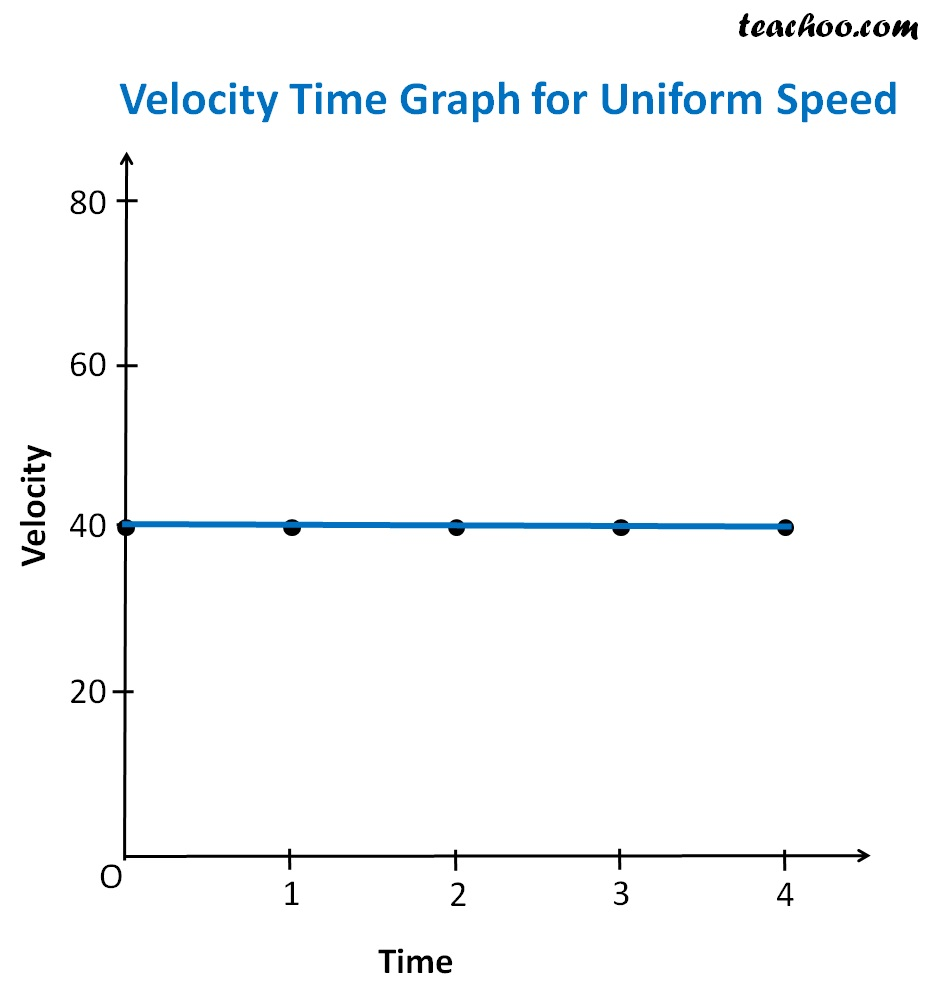Note

In this case,velocity time graph is in the form of Straight line parallel to x axis because speed of object does not change

(In our case,remains constant at 40 km/hour)

## Velocity-Time Graph for Uniform  Acceleration

Suppose an object travels at uniform acceleration of 20 km/hour

It means its speed is 20 km/hour in first hour,40 km/hour in second hour and 60 km /hour in third hour

So, his velocity will look like

 Hour Velocity 0 0 1 20 2 40 3 60 4 80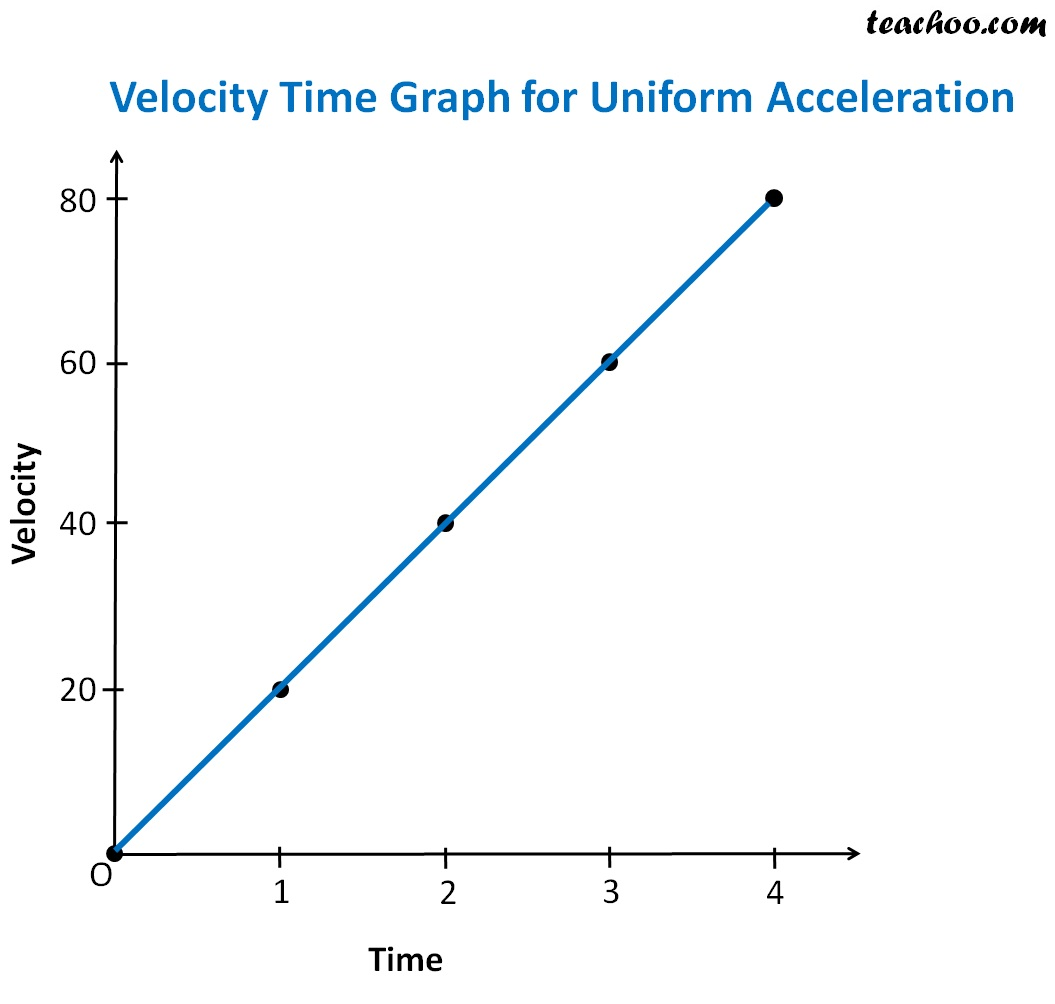Note
In this case,velocity time graph is in the form of Straight line sloping upwards

## Velocity-Time Graph for Non-Uniform  Acceleration

Suppose an object travels at a velocity of 5 km/hour in first hour, 25 km/hour in second hour, 60 km/hr in third hour and 80 km/hour in fourth hour

 Hour Velocity 0 0 1 5 2 25 3 60 4 80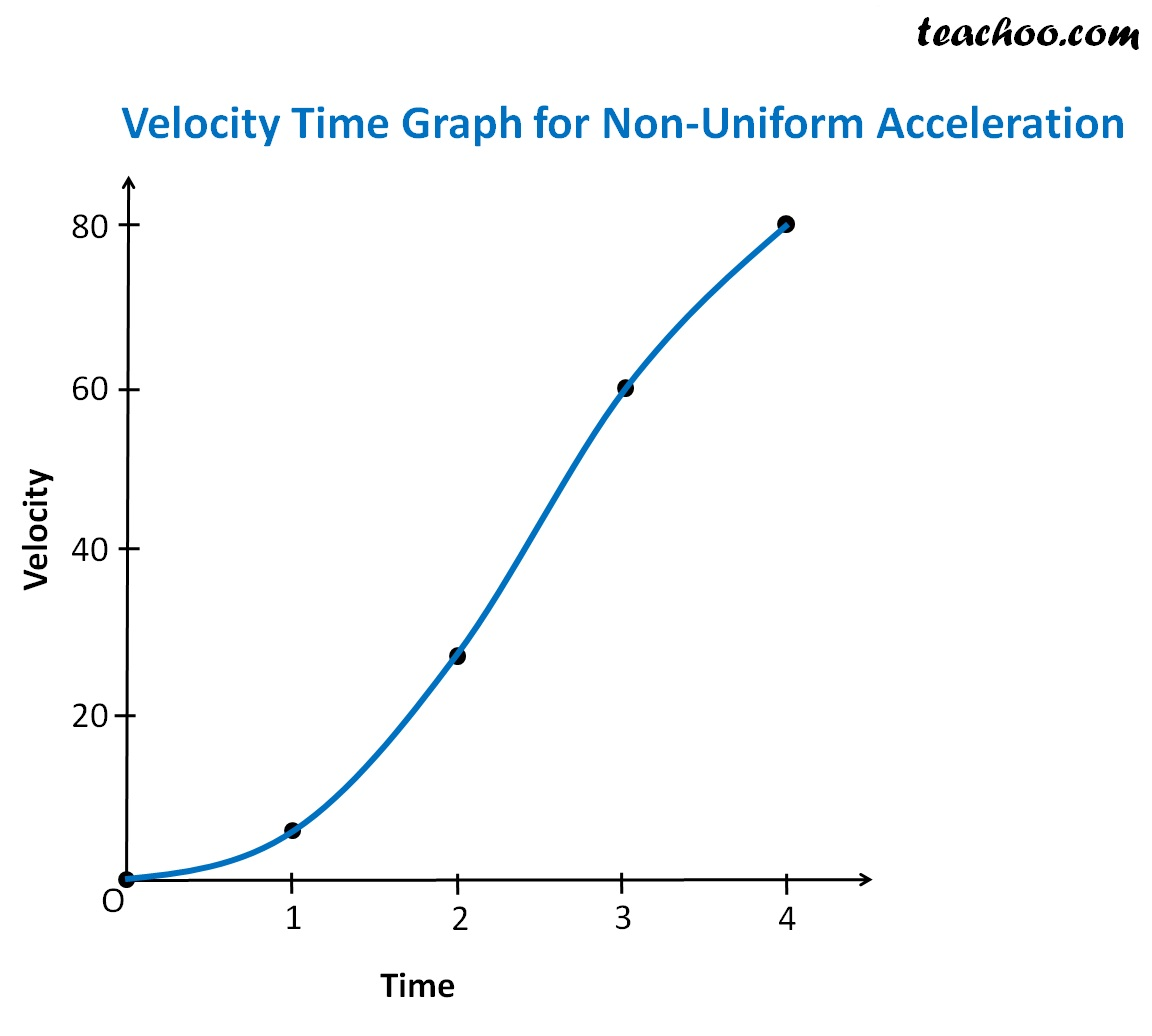Note
In this case,velocity time graph is in the form of Curved line sloping upwards

## Velocity-Time Graph for Uniform Retardation

Suppose an object travels at uniform retardation of 20 km/hour

If his speed is 80 km/hr in the beginning.

It means that its speed will be 60 km/hour in first hour, 40 km/hour in second hour and 20 km /hour in third hour

So, his velocity will look like

 Hour Velocity 0 80 1 60 2 40 3 20 4 0

## Velocity-Time Graph for Non-Uniform  Retardation

Sometimes velocity or speed of on object decreases

Suppose an object was traveling at a speed of 60 km/hour

its speed decreases to 40 km/hour in second hour and 20 km/hour in third year

 Hour Velocity 0 80 1 70 2 40 3 10 4 0

In this case,there is uniform retardation (or negative acceleration) of 20 km/hour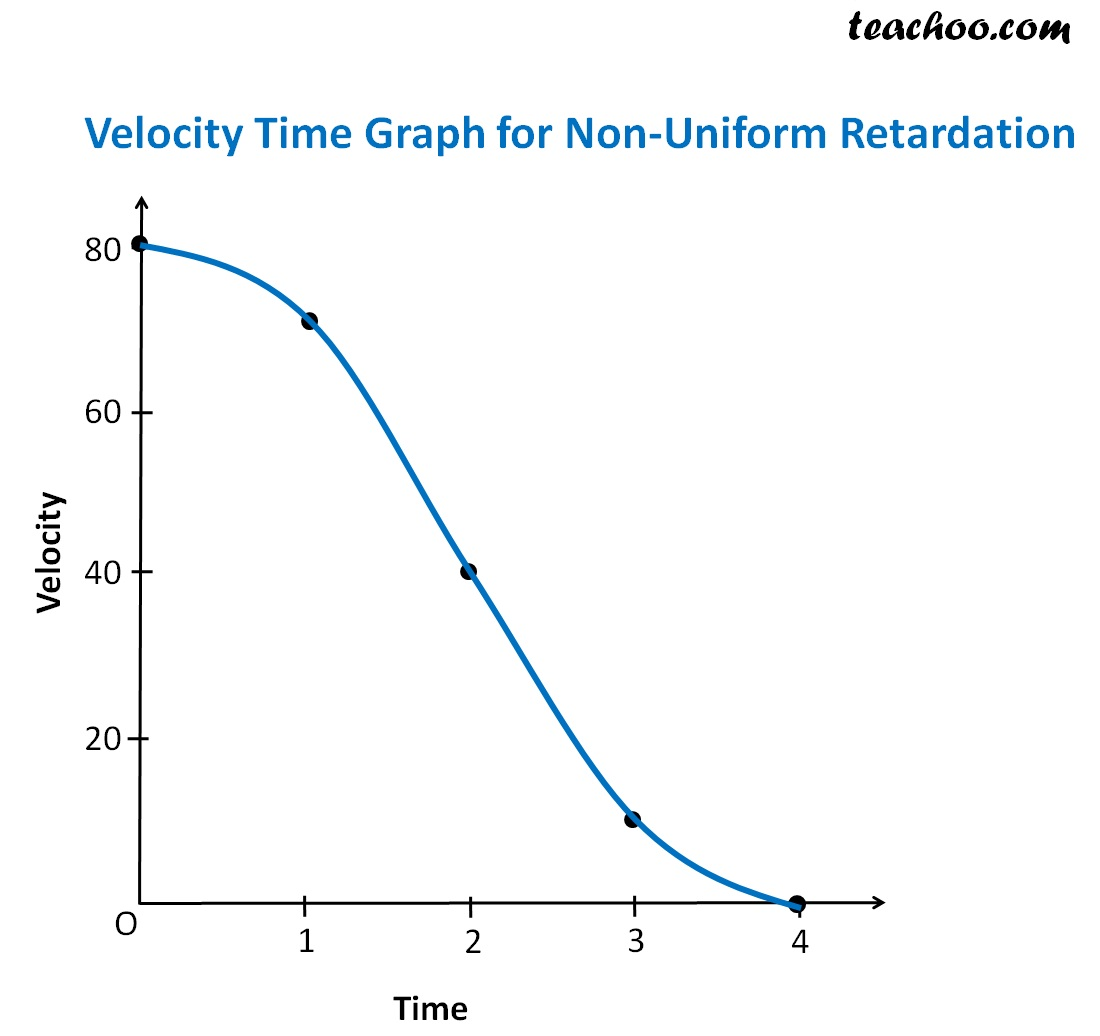So distance time graph is in form of a straight line sloping downwards

How to Find Acceleration and Distance by Velocity Time Graph

Acceleration = Slope of v-t graph

Distance = Area under v-t graph bounded by its axes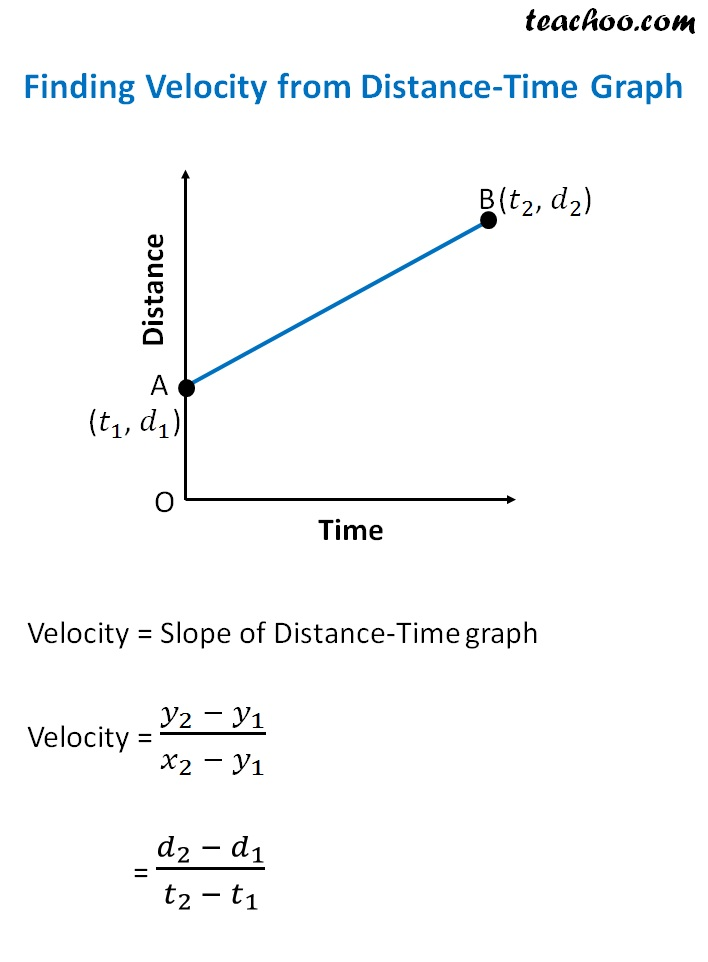How to find Distance travelled

## Velocity Time Graph Summary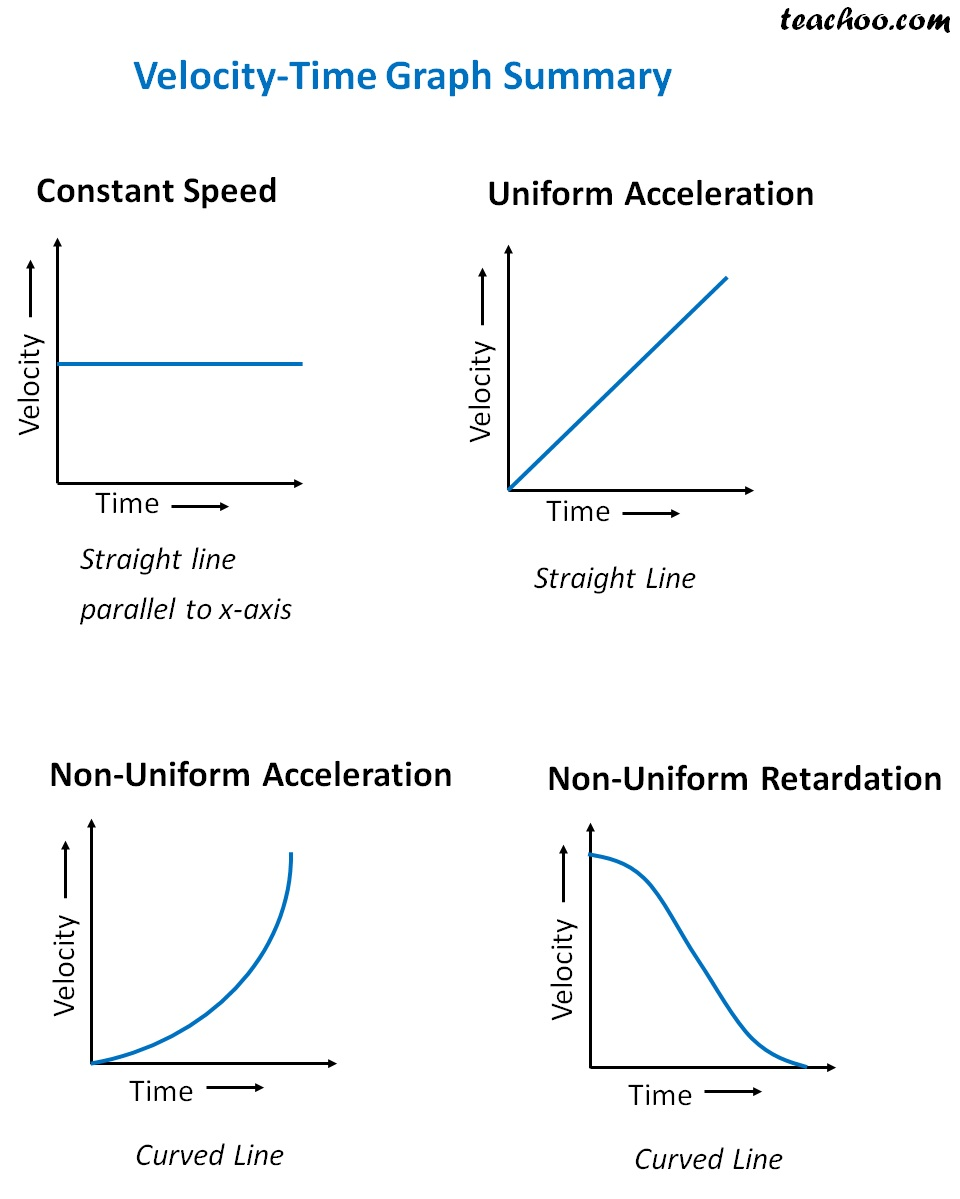## Questions

NCERT Question 5 - A driver of a car traveling at 52 km h–1 applies the brakes and accelerates uniformly in the opposite direction. The car stops in 5 s. Another driver going at 3 km h–1 in another car applies his brakes slowly and stops in 10 s. On the same graph paper, plot the speed versus time graphs for the two cars. Which of the two cars travelled farther after the brakes were applied?

NCERT Question 8 - The speed-time graph for a car is shown is Fig. 8.12.
(a) Find how far does the car travel in the first 4 seconds. Shade the area on the graph that represents the distance travelled by the car during the period.
(b) Which part of the graph represents uniform motion of the car?

Learn in your speed, with individual attention - Teachoo Maths 1-on-1 Class

### Transcript

Finding Acceleration from Velocity-Time Graph Acceleration = Slope of Velocity-Time graph Acceleration = (𝑦_2 − 𝑥_1)/(𝑥_2 − 𝑥_1 ) = (𝑣_2 − 𝑣_1)/(𝑡_2 − 𝑡_1 ) Finding Distance from Velocity-Time GraphDistance = Area of Velocity-Time graph Distance = Area of Trapezium OABC = 1/2 × Sum of Parallel Sides × Height = 1/2 × (OA + BC) × OC